<![CDATA[Advances in Applied Probability]]>https://www.cambridge.org/core/journals/advances-in-applied-probability/latest-issueAdvances in Applied Probability0001867814756064Cambridge University Press<![CDATA[Limit theorems for process-level Betti numbers for sparse and critical regimes]]>

The objective of this study is to examine the asymptotic behavior of Betti numbers of Čech complexes treated as stochastic processes and formed from random points in the d-dimensional Euclidean space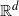. We consider the case where the points of the Čech complex are generated by a Poisson process with intensity nf for a probability density f. We look at the cases where the behavior of the connectivity radius of the Čech complex causes simplices of dimension greater thanto vanish in probability, the so-called sparse regime, as well when the connectivity radius is of the order of, the critical regime. We establish limit theorems in the aforementioned regimes: central limit theorems for the sparse and critical regimes, and a Poisson limit theorem for the sparse regime. When the connectivity radius of the Čech complex is, i.e. the sparse regime, we can decompose the limiting processes into a time-changed Brownian motion or a time-changed homogeneous Poisson process respectively. In the critical regime, the limiting process is a centered Gaussian process but has a much more complicated representation, because the Čech complex becomes highly connected with many topological holes of any dimension.

]]>
<![CDATA[Transient analysis for exponential time-limited polling models under the preemptive repeat random policy]]>

Polling systems are queueing systems consisting of multiple queues served by a single server. In this paper we analyze two types of preemptive time-limited polling systems, the so-called pure and exhaustive time-limited disciplines. In particular, we derive a direct relation for the evolution of the joint queue length during the course of a server visit. The analysis of the pure time-limited discipline builds on and extends several known results for the transient analysis of an M/G/1 queue. For the analysis of the exhaustive discipline we derive several new results for the transient analysis of the M/G/1 queue during a busy period. The final expressions for both types of polling systems that we obtain generalize previous results by incorporating customer routeing, generalized service times, batch arrivals, and Markovian polling of the server.

]]>
https://dx.doi.org/10.1017/apr.2019.51?rft_dat=source%3Ddrssdoi:10.1017/apr.2019.51Transient analysis for exponential time-limited polling models under the preemptive repeat random policy326010.1017/apr.2019.51https://dx.doi.org/10.1017/apr.2019.51?rft_dat=source%3Ddrss2020-04-29Advances in Applied Probability2020-04-29De Haan, RolandAl Hanbali, AhmadBoucherie, Richard J.Van Ommeren, Jan-Kees
<![CDATA[A non-exponential extension of Sanov’s theorem via convex duality]]>

This work is devoted to a vast extension of Sanov’s theorem, in Laplace principle form, based on alternatives to the classical convex dual pair of relative entropy and cumulant generating functional. The abstract results give rise to a number of probabilistic limit theorems and asymptotics. For instance, widely applicable non-exponential large deviation upper bounds are derived for empirical distributions and averages of independent and identically distributed samples under minimal integrability assumptions, notably accommodating heavy-tailed distributions. Other interesting manifestations of the abstract results include new results on the rate of convergence of empirical measures in Wasserstein distance, uniform large deviation bounds, and variational problems involving optimal transport costs, as well as an application to error estimates for approximate solutions of stochastic optimization problems. The proofs build on the Dupuis–Ellis weak convergence approach to large deviations as well as the duality theory for convex risk measures.

]]>
<![CDATA[An elementary derivation of moments of Hawkes processes]]>

Hawkes processes have been widely used in many areas, but their probability properties can be quite difficult. In this paper an elementary approach is presented to obtain moments of Hawkes processes and/or the intensity of a number of marked Hawkes processes, in which the detailed outline is given step by step; it works not only for all Markovian Hawkes processes but also for some non-Markovian Hawkes processes. The approach is simpler and more convenient than usual methods such as the Dynkin formula and martingale methods. The method is applied to one-dimensional Hawkes processes and other related processes such as Cox processes, dynamic contagion processes, inhomogeneous Poisson processes, and non-Markovian cases. Several results are obtained which may be useful in studying Hawkes processes and other counting processes. Our proposed method is an extension of the Dynkin formula, which is simple and easy to use.

]]>
<![CDATA[Thinning and multilevel Monte Carlo methods for piecewise deterministic (Markov) processes with an application to a stochastic Morris–Lecar model]]>

In the first part of this paper we study approximations of trajectories of piecewise deterministic processes (PDPs) when the flow is not given explicitly by the thinning method. We also establish a strong error estimate for PDPs as well as a weak error expansion for piecewise deterministic Markov processes (PDMPs). These estimates are the building blocks of the multilevel Monte Carlo (MLMC) method, which we study in the second part. The coupling required by the MLMC is based on the thinning procedure. In the third part we apply these results to a two-dimensional Morris–Lecar model with stochastic ion channels. In the range of our simulations the MLMC estimator outperforms classical Monte Carlo.

]]>
https://dx.doi.org/10.1017/apr.2019.55?rft_dat=source%3Ddrssdoi:10.1017/apr.2019.55Thinning and multilevel Monte Carlo methods for piecewise deterministic (Markov) processes with an application to a stochastic Morris–Lecar model13817210.1017/apr.2019.55https://dx.doi.org/10.1017/apr.2019.55?rft_dat=source%3Ddrss2020-04-29Advances in Applied Probability2020-04-29Lemaire, VincentThieullen, MichÉleThomas, Nicolas
<![CDATA[Simulation of elliptic and hypo-elliptic conditional diffusions]]>

Suppose X is a multidimensional diffusion process. Assume that at time zero the state of X is fully observed, but at time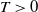only linear combinations of its components are observed. That is, one only observes the vectorfor a given matrix L. In this paper we show how samples from the conditioned process can be generated. The main contribution of this paper is to prove that guided proposals, introduced in , can be used in a unified way for both uniformly elliptic and hypo-elliptic diffusions, even when L is not the identity matrix. This is illustrated by excellent performance in two challenging cases: a partially observed twice-integrated diffusion with multiple wells and the partially observed FitzHugh–Nagumo model.

]]>
<![CDATA[Gumbel and Fréchet convergence of the maxima of independent random walks]]>

We consider point process convergence for sequences of independent and identically distributed random walks. The objective is to derive asymptotic theory for the largest extremes of these random walks. We show convergence of the maximum random walk to the Gumbel or the Fréchet distributions. The proofs depend heavily on precise large deviation results for sums of independent random variables with a finite moment generating function or with a subexponential distribution.

]]>
<![CDATA[Joint temporal and contemporaneous aggregation of random-coefficient AR(1) processes with infinite variance]]>

We discuss the joint temporal and contemporaneous aggregation of N independent copies of random-coefficient AR(1) processes driven by independent and identically distributed innovations in the domain of normal attraction of an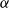-stable distribution,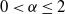, as both N and the time scale n tend to infinity, possibly at different rates. Assuming that the tail distribution function of the random autoregressive coefficient regularly varies at the unit root with exponent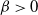, we show that, for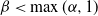, the joint aggregate displays a variety of stable and non-stable limit behaviors with stability index depending on,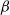and the mutual increase rate of N and n. The paper extends the results of Pilipauskaitė and Surgailis (2014) from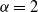to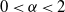.

]]>
https://dx.doi.org/10.1017/apr.2019.59?rft_dat=source%3Ddrssdoi:10.1017/apr.2019.59Joint temporal and contemporaneous aggregation of random-coefficient AR(1) processes with infinite variance23726510.1017/apr.2019.59https://dx.doi.org/10.1017/apr.2019.59?rft_dat=source%3Ddrss2020-04-29Advances in Applied Probability2020-04-29Pilipauskaitė, VytautėSkorniakov, ViktorSurgailis, Donatas
<![CDATA[The existence of a giant cluster for percolation on large Crump–Mode–Jagers trees]]>

In this paper we consider random trees associated with the genealogy of Crump–Mode–Jagers processes and perform Bernoulli bond-percolation whose parameter depends on the size of the tree. Our purpose is to show the existence of a giant percolation cluster for appropriate regimes as the size grows. We stress that the family trees of Crump–Mode–Jagers processes include random recursive trees, preferential attachment trees, binary search trees for which this question has been answered by Bertoin , as well as (more general) m-ary search trees, fragmentation trees, and median-of-(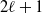) binary search trees, to name a few, where to our knowledge percolation has not yet been studied.

]]>
<![CDATA[On the multi-state signatures of ordered system lifetimes]]>

In this paper, the signature of a multi-state coherent system with binary-state components is discussed, and then it is extended to the case of ordered system lifetimes arising from a life-test on coherent multi-state systems with the same multi-state system signature. Some properties of the multi-state system signature and the ordered multi-state system signature are also studied. The results established here are finally explained through some illustrative examples.

]]>